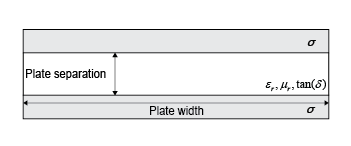# txlineParallelPlate

Create parallel-plate transmission line

Since R2020b

## Description

Use the `txlineParallelPlate` object to create a parallel-plate transmission line. A cross-section of a parallel-plate transmission line is shown this figure. The physical characteristics of the parallel-plate transmission line include the plate width and the plate separation.## Creation

### Syntax

``paralleltxline = txlineParallelPlate``
``paralleltxline = txlineParallelPlate(Name,Value)``

### Description

````paralleltxline = txlineParallelPlate` creates a parallel-plate transmission line object.```

example

````paralleltxline = txlineParallelPlate(Name,Value)` sets properties using one or more name-value pairs. For example, ```txline = txlineParallelPlate('Separation',0.0046)``` creates a parallel-plate transmission line with a separation of `0.0046` meters.```

## Properties

expand all

Name of the parallel-plate transmission line, specified as a string scalar or a character vector.

Example: `'Name','parallelplate1'`

Example: `paralleltxline.Name = 'parallelplate1'`

Data Types: `char` | `string`

Physical length of the transmission line, specified as a positive scalar in meters.

Example: `'LineLength',0.0200`

Example: `paralletxline.LineLength = 0.0200`

Data Types: `double`

Physical width of the transmission line, specified as a positive scalar in meters.

Example: `'Width',0.00200`

Example: `paralleltxline.Width = 0.00200`

Data Types: `double`

Thickness of dielectric, specified as a positive scalar in meters.

Example: `'Separation',0.007`

Example: `paralleltxline.Separation = 0.007`

Data Types: `double`

Relative permeability of a dielectric, specified as a positive scalar.

The ratio of permeability of dielectric, μ, to the permeability in free space, μ0.

Example: `'MuR',1.5`

Example: `paralleltxline.MuR = 1.5`

Data Types: `double`

Relative permittivity of the dielectric, specified as a positive scalar in Farad per meter (F/m).

Example: `'EpsilonR',3.3`

Example: `paralleltxline.EpsilonR = 3.3`

Data Types: `double`

Loss angle tangent of the dielectric, specified as a nonnegative scalar.

Example: `'LossTangent',1`

Example: `paralleltxline.LossTangent = 1`

Data Types: `double`

Conductivity of the conductor, specified as a positive scalar in Siemens per meter (S/m).

Example: `'SigmaCond',2`

Example: `paralleltxline.SigmaCond = 2`

Data Types: `double`

Stub transmission line termination, specified as `'NotApplicable'`, `'Open'`, or `'Short'`.

Example: `'Termination','Short'`

Example: `paralleltxline.Termination = 'Short'`

Data Types: `char`

Type of stub, specified as `'NotAStub'`, `'Series'`, or `'Shunt'`.

Example: `'StubMode','Series'`

Example: `paralleltxline.StubMode = 'Series'`

Data Types: `char`

Number of input and output ports, specified as positive scalar.

Data Types: `double`

Terminals of coaxial transmission line, specified as a cell array of strings.

Data Types: `char` | `string`

## Object Functions

 `sparameters` Calculate S-parameters for RF data, network, circuit, and matching network objects `groupdelay` Group delay of S-parameter object or RF filter object or RF Toolbox circuit object `noisefigure` Calculate noise figure of transmission lines, series RLC, and shunt RLC circuits `getZ0` Calculate characteristic impedance with and without dispersion for transmission line `circuit` Circuit object `clone` Create copy of existing circuit element or circuit object

## Examples

collapse all

Create a parallel-plate transmission line using these specifications:

• Width : 300 mm

• Separation between plates : 10 mm

• EpsilonR : 4.2 F/m

`parallelplatetxline = txlineParallelPlate('Width',300e-3,'Separation',10e-3,'EpsilonR',4.2)`
```parallelplatetxline = txlineParallelPlate: ParallelPlate element Name: 'ParallelPlate' Width: 0.3000 Separation: 0.0100 MuR: 1 EpsilonR: 4.2000 LossTangent: 0 SigmaCond: Inf LineLength: 0.0100 Termination: 'NotApplicable' StubMode: 'NotAStub' NumPorts: 2 Terminals: {'p1+' 'p2+' 'p1-' 'p2-'} ```

Calculate the S-parameters of the transmission line at 6 GHz.

`sparam = sparameters(parallelplatetxline,6e9);`

## Version History

Introduced in R2020b